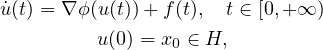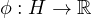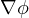Electron. J. Differential Equations, Vol. 2021 (2021), No. 15, pp. 1-13.

### Asymptotic behavior for a quasi-autonomous gradient system of expansive type governed by a quasiconvex function Behzad Djafari Rouhani, Mohsen Rahimi Piranfar

Abstract:
We consider the quasi-autonomous first-order gradient systemwhereis a differentiable quasiconvex function such thatis Lipschitz continuous. We study the asymptotic behavior of solutions to this system in continuous and discrete time. We show that each solution either approaches infinity in norm or converges weakly to a critical point of φ. This further concludes that the existence of bounded solutions and implies that φ has a nonempty set of critical points. Some strong convergence results, as well as numerical examples, are also given in both continuous and discrete cases.

Submitted January 25, 2021. Published March 18, 2021.
Math Subject Classifications: 34G20, 47J35, 39A30, 37N40.
Key Words: First-order evolution equation; expansive type gradient system; asymptotic behavior; quasiconvex function; minimization.
DOI: https://doi.org/10.58997/ejde.2021.15

Show me the PDF file (325 KB), TEX file for this article.Behzad Djafari Rouhani Department of Mathematical Sciences University of Texas at El Paso 500 W. University Ave., El Paso, TX 79968, USA email: behzad@utep.edu Mohsen Rahimi Piranfar Isfahan Mathematics House Isfahan, Iran email: m.piranfar@gmail.com